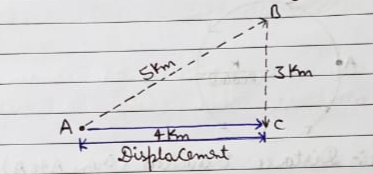5/5 - (1 vote)

# Motion Class 9 Notes Science Chapter 8

Stationary
Object
:-The object
which remains Fixed at a place is called Stationary object.

E.g:-Trees, a house, a school, a factory

Moving
Object:-
The object
which can move from one place to another is called moving object.

E.g:- a man moves when he walks along a
road, a bird moves when it flies in   the
sky etc….

Note:- A common characterstics of all the
moving bodies is that they change their position with time.

Reference
Point:-
A body is
said to be in motion (or moving) when its position changes contiously with
respect to a stationay object taken as reference point.

Note:-If a body moves fairly fast, then
its movement(or motion) can be observed easily. But if a body moves very
slowly, the it become difficult to observe its movement immediately.

E.g:- A wrist watch has
three hands:- a second hand, a minute hand and an hour hand, which moves round
and round on the dial of the watch. Now, the seconds’ hand of watch moves quite
fast, so we can observe the movement of the second hand of watch very easily.
But the minutes hand and an hour hand of a watch moves quite slowly, so their
movement cannot be observed easily.

Motion:- The body is said to be in motion
when its position changes continuously with respect to time.

Note:- Physical quantity:- The quantity
which can be measured or quantities of Physics

E.g. The quantity like distance,
displacement, etc. are known as physical quantity.

Scalar
Quantity:-
A
physical quantity having only magnitude( or size) is known as a scalar
quantity.

E.g:- Distance, speed, mass, length, time

Vector
Quantity:-
A
physical quantity having magnitude as well as direction is called vector
quantity.

E.g:- Displacement, velocity,
acceleration, force, moventum, torque, Angular movementum

## Distance Travelled and Displacement

Distance(‘D’):- The distance travelled by a body is
the actual length of the path covered by a moving body irrespective of the
direction in which the body travels.

E.g:- In this case, the actual length of
the path covered by the man is 5km+3km=8km, so the distance travelled by the
man is 8km.Displacement(“s”):-When a body moves from one position
to another, the shortest(straight line) distance between the initial position
and final position of the body, along with direction, is known as its
displacement.
Eg:- In this case, the shortest distance between the initial position “A” and Final postion “C” of the man is 4 km, so the displacement pf man is 4km in the east direction.
Note;- The distance travelled by a moving body cannot be zero but the final displacement of a moving body can be zero.
The displacement of a moving body will be zero if, after travelling a certain distance, the moving body finally comes back to its stating position.

Note:- If we a round trip and reach back at the starting point then, though we have travelled some distance, our final displacement will be zero. This is because the straight line distance between the initial and final position will be zero.

Note:- Whenever a body travels along a zig-zag path, the final displacement is obtained by joining the starting point and finishing point of the body by straight line.

## Uniform Motion And Non-Uniform Motion

1. Uniform Motion:- A body has a uniform if it travels equal distances in equal interval of time, no matter how small these time interval may be.
2. Non-uniform Motion:- A body has a non-uniform motion if it travels unequal distance in equal interval of time, no matter how small these time interval may be.
Notes:- If the distance-time graph is a straight line, the motion will be uniform and if the distance-time graph is curved line, motion will be non-uniform.